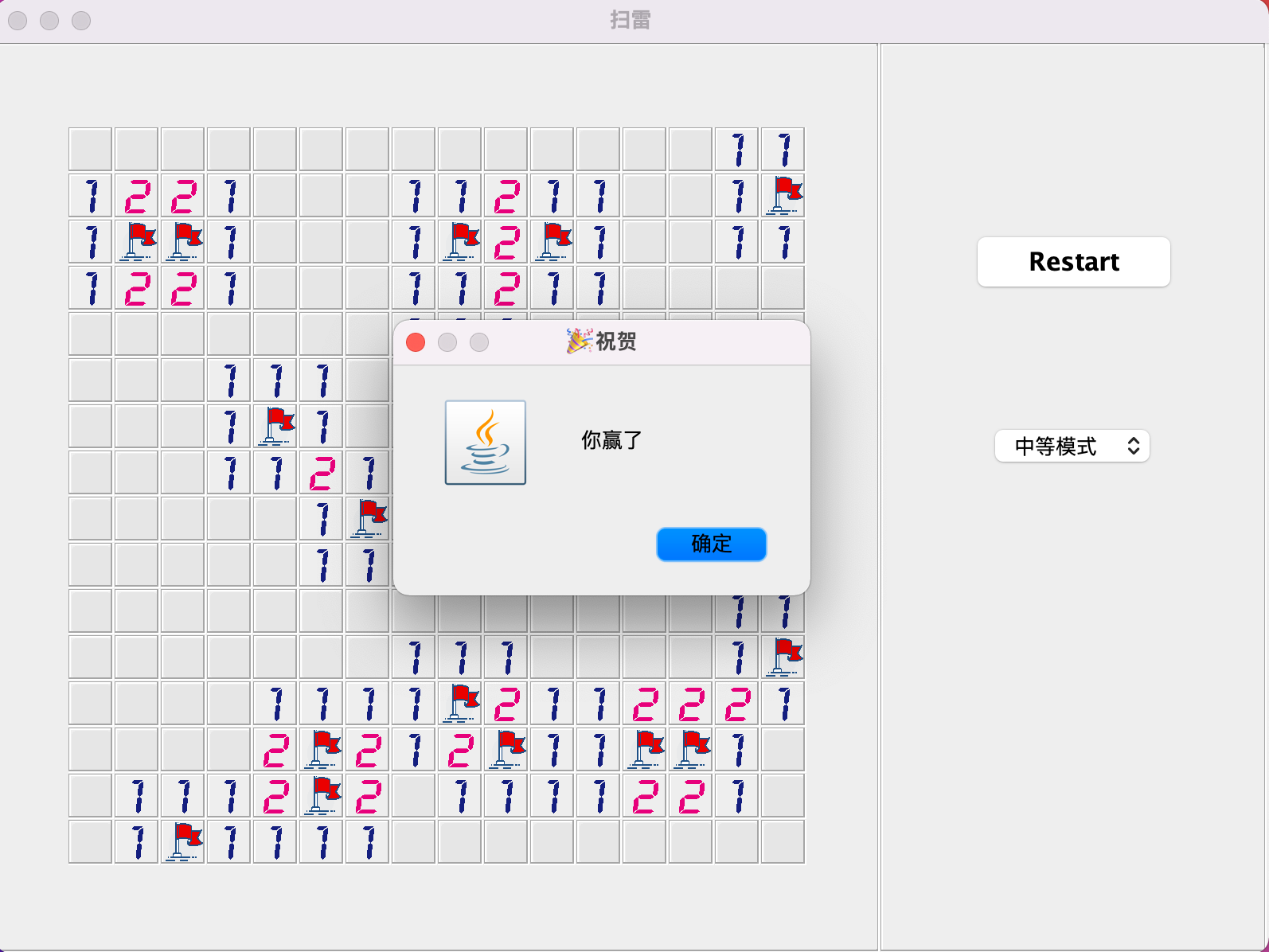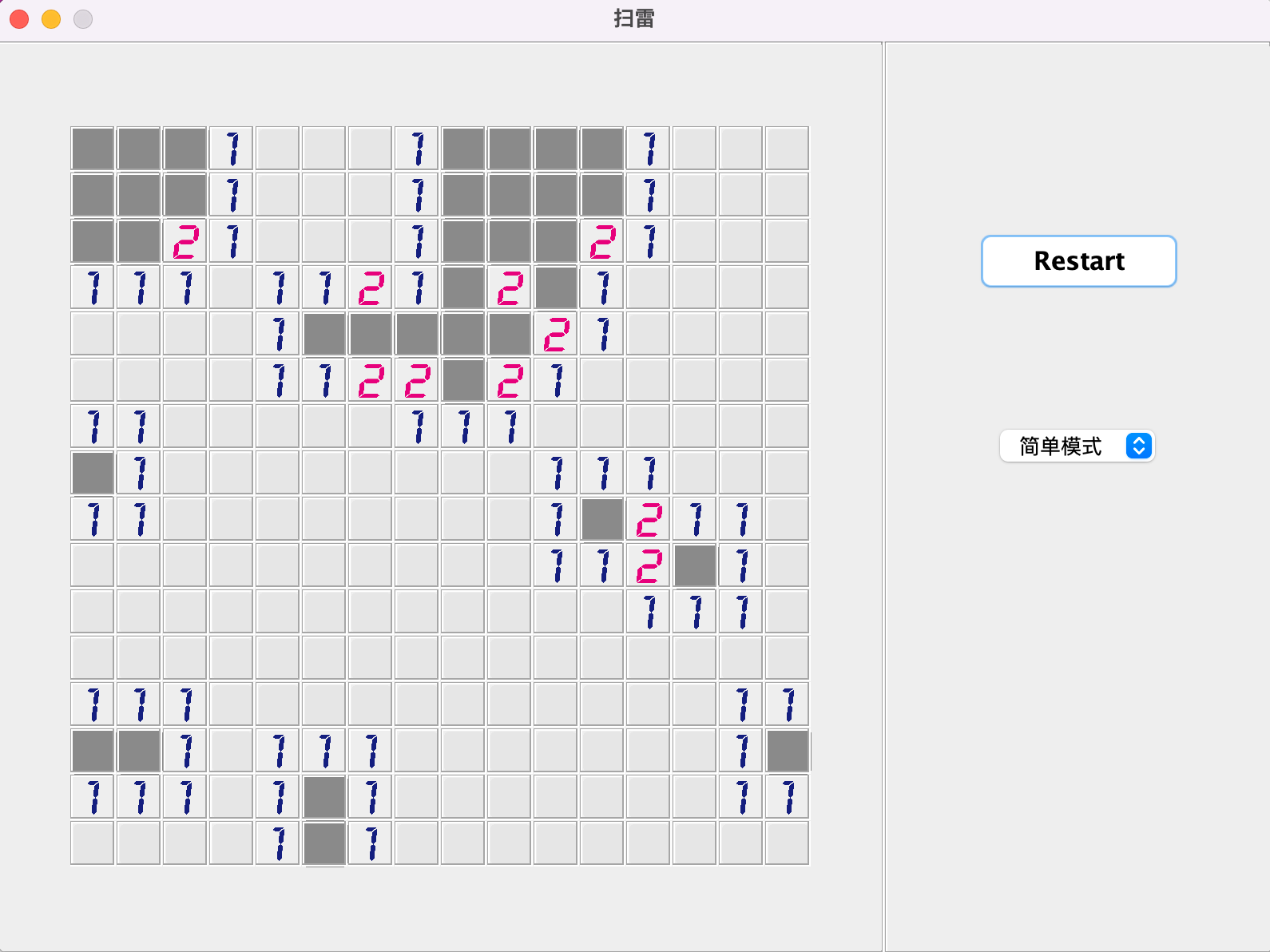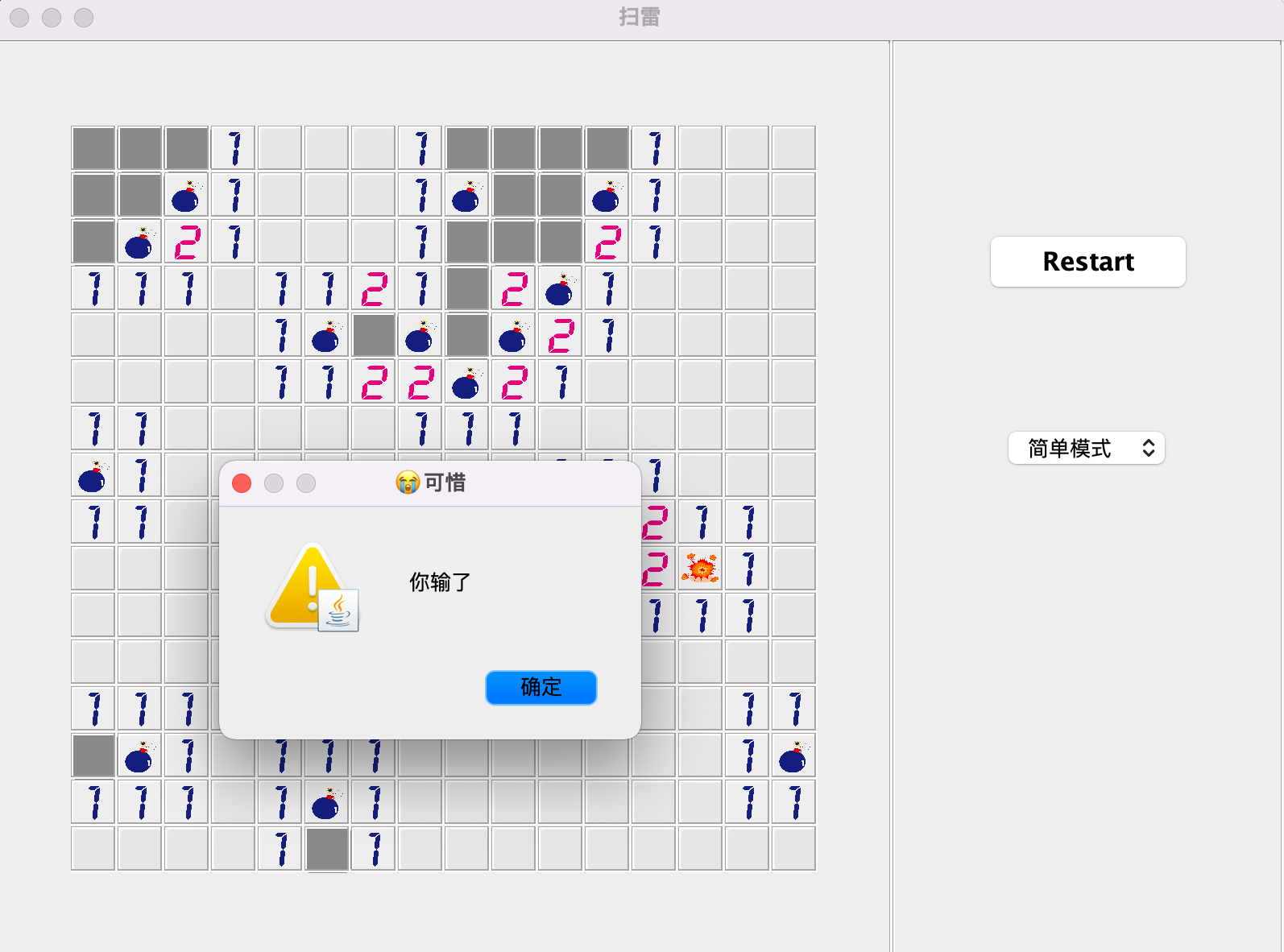• 累计撰写 141 篇文章
• 累计创建 37 个标签
• 累计收到 11 条评论

无聊的周末用Java写个扫雷小游戏2022-01-11 / 0 评论 / 0 点赞 / 26 阅读 / 3,834 字

Swing 是过时了，但是好玩不会过时，不喜勿喷• 数据结构设计
• 视图和数据尽可能分开
• 点击时候使用BFS扫描
• 判断成功失败

数据结构设计

public class Data {
// 游戏状态
// 雷区大小
public static int size = 16;
// 雷的数量
public static int numOfMine = 0;
// 表示是否有雷，1：有，0没有
public static int[][] maps = null;
// 是否被访问
public static boolean[][] visited = null;
// 周边雷的数量
public static int[][] nums = null;
// 是否被标记
public static boolean[][] flags = null;
// 上次被访问的块坐标
public static Point lastVisitedPoint = null;
// 困难模式
private static DifficultModeEnum mode;
...
}

• 游戏状态：是否开始，结束，成功，失败等等
• 模式：简单，中等或者困难，这个会影响自动生成的雷的数量
• 雷区的大小：16*16的小方块
• 雷的数量：与模式选择有关，是个随机数
• 标识每个方块是否有雷：最基础的数据，生成之后需要同步更新这个数据
• 标识每个方块是否被扫过：默认没有扫过
• 每个方块周边类雷的数量：生成的时候同步计算该结果，不想每次点击后再计算，毕竟是个不会更新的数据，一劳永逸
• 标识方块是否被标记：扫雷的时候我们使用小旗子标记方块，表示这里是雷，标识完所有的雷的时候，成功
• 上次访问的方块坐标：这个其实可以不记录，但是为了表示爆炸效果，与其他的雷展示不一样，故而记录下来

视图与数据分开new MouseListener() {
@Override
public void mouseClicked(MouseEvent e) {
if (Data.status == Status.GOING) {
int c = e.getButton(); // 得到按下的鼠标键
Block block = (Block) e.getComponent();
int x = block.getPoint_x();
int y = block.getPoint_y();
if (c == MouseEvent.BUTTON1) {
Data.visit(x, y);
} else if (c == MouseEvent.BUTTON3) {// 推断是鼠标右键按下
if (!Data.visited[x][y]) {
Data.flags[x][y] = !Data.flags[x][y];
}
}
}
repaintBlocks();
}
}

public class Block extends JPanel {
private int point_x;
private int point_y;

private String backgroundPath = ImgPath.DEFAULT;

public Block(int x, int y) {
this.point_x = x;
this.point_y = y;
setBorder(BorderFactory.createEtchedBorder());
}
}

@Override
protected void paintComponent(Graphics g) {
refreshBackground();
ImageIcon icon = new ImageIcon(url);
if (backgroundPath.equals(ImgPath.DEFAULT) || backgroundPath.equals(ImgPath.FLAG)
|| backgroundPath.equals(String.format(ImgPath.NUM, 0))) {
g.drawImage(icon.getImage(), 0, 0, getWidth(), getHeight(), this);
} else {
int x = (int) (getWidth() * 0.1);
int y = (int) (getHeight() * 0.15);
g.drawImage(icon.getImage(), x, y, getWidth() - 2 * x, getHeight() - 2 * y, this);
}
}

BFS扫描

BFS，也称为广度优先搜索，这算是扫雷里面的核心知识点，也就是点击的时候，如果当前方块是空的，那么就会触发扫描周边的方块，同时周边方块如果也是空的，会继续递归下去，我用了广度优先搜索，也就是先将它们放到队列里面，取出来，再判断是否为空，再将周边符合的方块添加进去，进行一一处理。

public static void visit(int x, int y) {
lastVisitedPoint.x = x;
lastVisitedPoint.y = y;
if (maps[x][y] == 1) {
status = Status.FAILED;
// 游戏结束，暴露所有的雷
} else {
// 点击的不是雷
while (!points.isEmpty()) {
Point point = points.poll();
visited[point.x][point.y] = true;
if (nums[point.x][point.y] == 0) {
}
}
}
}

public static void addToVisited(Queue<Point> points, int i, int j) {
int x = i - 1;
while (x <= i + 1) {
if (x >= 0 && x < size) {
int y = j - 1;
while (y <= j + 1) {
if (y >= 0 && y < size) {
if (!(x == i && j == y)) {
// 没访问过且不是雷
if (!visited[x][y] && maps[x][y] == 0) {
}
}
}
y++;
}
}
x++;
}
}判断成功失败【作者简介】

0